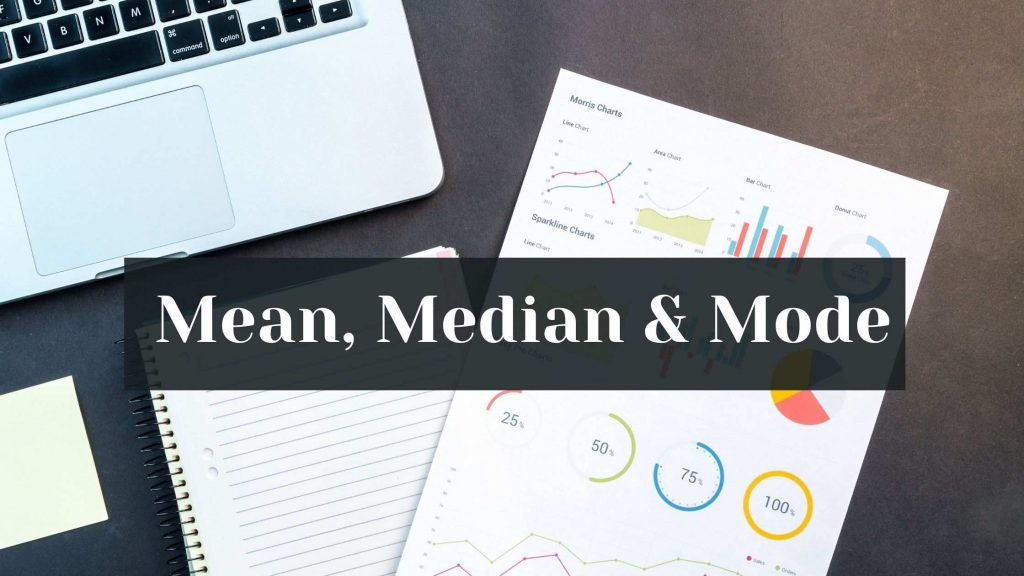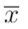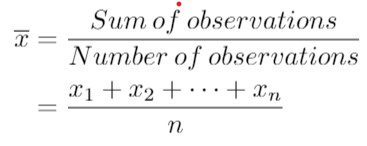Maths
Study Material

# Mean, Median, Mode-Introduction

In this blog, we are going to find out what mean, median and mode of data represent and how they are useful.

3 minutes long
Posted by Tanwir Silar, 18/8/2021Hesap Oluştur

Got stuck on homework? Get your step-by-step solutions from real tutors in minutes! 24/7. Unlimited.

Mean is the most heard measure of central tendency. Mode and median are the other two measures of central tendency. In this blog, let’s learn mean, median, mode and their applications

## Mean

Mean is the most referred measure of central tendency. It is obtained by evaluating the sum of all the values in the data and dividing the resultant sum by the number observations in the data. It is also known as average.
Usually, represented by the symbol(read as x bar).
Note: When there are outliers in the data, mean gets affected by them as it has to include all of the observations of the data.

If the data consists of n observations x1, x2, … , xn, then the mean of the data isExample 1:
Find the mean of the following discrete data: 34, 73, 42, 81, 17.
Here the number of observations = n = 5.
Sum of the observations = 34 + 73 + 45 + 81 + 17 = 250.
Mean = Sum/n = 250/5 = 50.

## Median

The value of median is obtained by finding the middle element when the data is sorted in either ascending order or descending order. In case of outliers in the data, median comes handy as it isn’t affected by the outliers in the data.

When there are odd number of observations, there is a single middle element which is present at (n+1)/2 position, and it is the median. Whereas, when there are even number of observations, there are two middle elements. Median is taken to be the average value of the two middle elements present at n/2 and n/2 +1 positions.

Example 2:
Find the median of the following data: 32, 4, 15, 91, 6, 52, 28.
Solution:
Initially, we need to sort the observations either in ascending order or descending order. Let us sort the observations in ascending order. The sequence then becomes, 4, 6, 15, 28, 32, 52, 91.
The number of observations here is 7. It means that number at (7+1)/2 = 4th position is the median.
The median of the data = 28.

Example 3:
Find the median of the following data: 65, 83, 25, 16, 97, 0.
Solution:
Sorting the numbers in ascending order, we get, 0, 16, 25, 65, 83, 97. The number of observations in the data = 6. Here, median is the average of the elements present at 3rd and 4th position.
Average of 25, 65 = (25+65)/2 = 90/2 = 45.

## Mode

The definition of mode is plain and clear. The mode is the most frequently occurring observation in the data. In general, sorting the observations helps in finding the mode.
Mode helps us to understand the data in terms of frequencies, as it’s value tells us about the observation appearing maximum number of times.

Example 4:
Find the mode of the following data: 2, 3, 6, 2, 1, 7, 2 19, 7, 4, 2, 5, 9, 0.
Solution:
Sorting the observations in ascending order to get, 0, 1, 2, 2, 2, 2, 3, 4, 5, 6, 7, 7, 9, 19.
Since, 2 has appeared 4 times (the highest by any observation), it is the mode of the data.

We hope, this blog(mean, median, mode) was helpful.